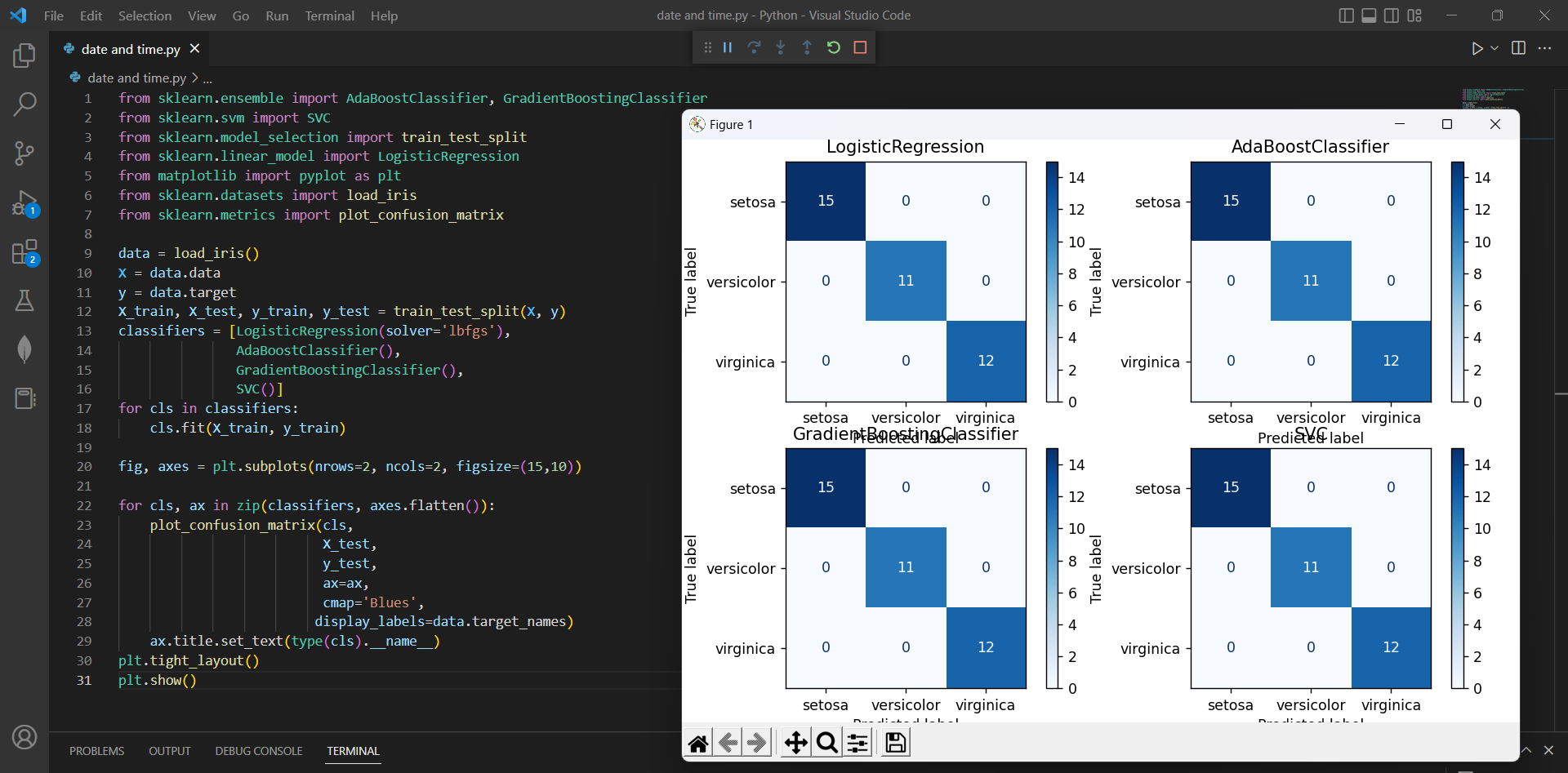# How to Plot Multiple confusion Matrices using Sk-Learnby vigneshchennai74Updated: Apr 6, 2023Solution Kit

Plotting multiple confusion matrices is useful for evaluating and comparing the performance of different machine-learning models.

In scikit-learn, you can plot multiple confusion matrices using the plot_confusion_matrix function. This function takes a trained classifier object, and the test data as inputs, and it plots a confusion matrix that shows the classifier's performance on the test data. Plotting multiple confusion matrices can be useful in several ways:

• Comparison of models: By plotting the confusion matrices of multiple models, you can compare their performance on the same dataset.
• Model selection: If you are still determining which model to choose for your problem, you can compare multiple models by plotting their confusion matrices. This can give you a visual representation of their performance and help you decide which model to use.
• Model evaluation: Confusion matrices provide a simple and effective way to evaluate the performance of a model. By plotting multiple confusion matrices, you can get a clearer picture of the strengths and weaknesses of different models and see how they compare in terms of overall accuracy, false positives, false negatives, and other metrics.
• Model improvement: By comparing the confusion matrices of different models, you can identify areas where models can be improved.

This code uses various machine learning algorithms from the sci-kit-learn library to classify the iris dataset. The iris dataset is loaded using the load_iris() function from sklearn.datasets module. plt.subplots is a function in the matplotlib library that creates a figure and multiple subplots within it. The function returns a figure and an array of subplots, which can be used to create multiple plots within the same figure.Fig1.Preview of the output that you will get on running this code from your IDE

1. Copy the code using the "Copy" button above, and paste it in a Python file in your IDE.
2. Run the file to plot multiple confusion matrix in single page

I hope you found this useful. I have added the link to dependent libraries, version information in the following sections.

I found this code snippet by searching for "Plot multiple confusion matrices with plot_confusion_matrix" in kandi. You can try any such use case!

### Dependent Library

scikit-learnby scikit-learn

Python54584Version:1.2.2scikit-learn: machine learning in Python

Support
Quality
Security
Reuse

scikit-learnby scikit-learn

Python54584Version:1.2.2License: Permissive (BSD-3-Clause)

scikit-learn: machine learning in Python
Support
Quality
Security
Reuse

If you do not have Scikit-learn that is required to run this code, you can install it by clicking on the above link and copying the pip Install command from the Scikit-learn page in kandi.

You can search for any dependent library on kandi like Scikit-learn.

### Environment Tested

I tested this solution in the following versions. Be mindful of changes when working with other versions.

1. The solution is created in Python 3.7.15 version
2. The solution is tested on scikit-learn 1.0.2 version

Using this solution, we are able to plot multiple confusion matrix using Scikit learn library in Python with simple steps. This process also facilities an easy to use, hassle free method to create a hands-on working version of code which would help us plot a confusion matrix in Python.

### Support

1. For any support on kandi solution kits, please use the chat
2. For further learning resources, visit the Open Weaver Community learning page.

See similar Kits and Libraries